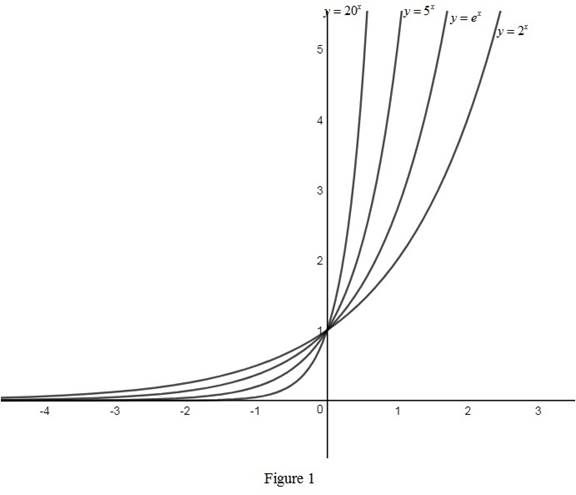# The graphs of the function y = 2 x , y = e x , y = 5 x and y = 20 x on the same axes and interpret how these graphs are related.### Single Variable Calculus: Concepts...

4th Edition
James Stewart
Publisher: Cengage Learning
ISBN: 9781337687805### Single Variable Calculus: Concepts...

4th Edition
James Stewart
Publisher: Cengage Learning
ISBN: 9781337687805

#### Solutions

Chapter 1.5, Problem 7E
To determine

## To sketch: The graphs of the function y=2x,y=ex,y=5x and y=20x on the same axes and interpret how these graphs are related.

Expert Solution

### Explanation of Solution

The rough graphs of the functions y=2x,y=ex,y=5x and y=20x are drawn and shown below in Figure 1.From Figure 1, it is observed that the graphs of the functions are increasing and passing through the common point (0, 1). Also, notice that the given functions are exponential functions.

Thus, it can be concluded that the graphs are increasing exponential functions whose base is greater than 1.

### Have a homework question?

Subscribe to bartleby learn! Ask subject matter experts 30 homework questions each month. Plus, you’ll have access to millions of step-by-step textbook answers!